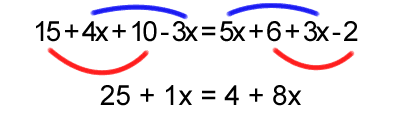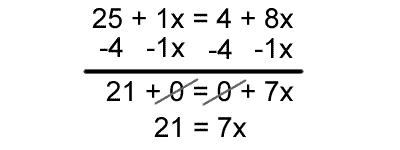# Combine Like Terms

A frequently-used procedure in algebra is the process of combining like terms. This is a way to "clean-up" an equation and make it much easier to solve. In case you've forgotten, a term is each single part of an expression. For example, in the expression $$4x + 3 + 7y$$, there are three terms: $$4x$$, $$3$$, and $$7y$$. The number 4 by itself is not a term, but rather a factor of the term $$4x$$.Let's say we are given the equation below. It looks very complicated, but if we look carefully, everything is either a constant (a number), or the variable x with a coefficient (4x). By the way, a coefficient is the number by which a variable is being multiplied (the 4 in 4x is the coefficient).

$$15+4x+10-3x = 5x+6+3x-2$$

The "like terms" in the equation above are ones that have the same variable. All constants are like terms as well. This means that the 15, 10, 6, and -2 are all one set of like terms, and the other is 4x, -3x, 5x, and 3x. To combine them is pretty easy, you just add them together and make sure that they are all on the same side of the equation. First, we will combine all like terms on each side of the equation:Since the 15 and 10 are both constants we combine them to get 25. The 4x and -3x each have the same variable (x), so we can add them to get 1x. Doing the same on the other side we arrive at 25 + 1x = 4 + 8x. The process is still not finished, however. There are still some like terms, but they are on opposite sides of the equal sign. Since we can do the same thing to both sides we just subtract 4 from each side and subtract 1x from each side:Now it's just a simple process of dividing by seven on each side:And we arrive at our answer of x=3. Combining like terms enabled you to take that huge mess of an equation and make it into something much more obvious. If you still are not sure about combining like terms, or are interested in more information, please visit our message board. Alternatively you can try another lesson on combining like terms from AlgebraHelp.com, or this lesson from Math.com.

### Summary

Combine terms with the same variable name to simplify an expression.